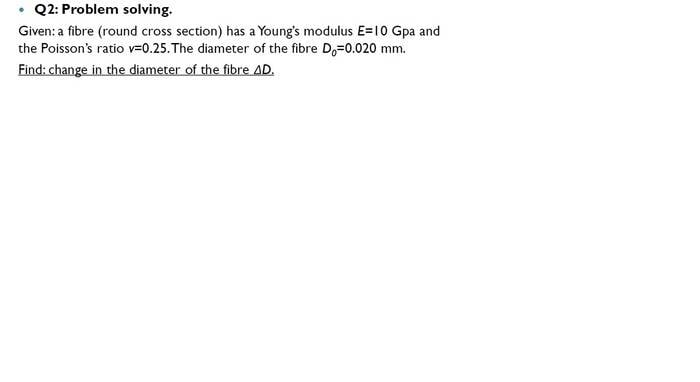# Question about stress and strain of a fiber

## Homework Statementi can not find the N.
what i can only found is:
D0=0.02mm;V=0.25;E=10G Pa;A=π(0.02mm/2)2
ΔD=εshear×D0
εshearaxial×V
εaxialaxial / E
σaxial=N/A

## Answers and Replies

haruspex
Science Advisor
Homework Helper
Gold Member
2020 Award
I don't see anything in the question about a tension being applied, so why should it change diameter?

•Chestermiller
stressShear= E×I
R=0.01mm,E=10GPa, I0= (π/4)×(0.01×10(-3))4=7.85 zm4
Stressshear=(10×109×7.85×10-21)
=78.5p Pa

Shear modulus=E/[2(1+V)] = 10G/[2(1+0.25)]=4GPa
Shear modulus= Stressshearshear
εshear=78.5p/4G=19.625z

ΔD=εshear×D0
=19.625z×0.02m
=0.3925y m
Is it right?

haruspex
Science Advisor
Homework Helper
Gold Member
2020 Award
stressShear= E×I
R=0.01mm,E=10GPa, I0= (π/4)×(0.01×10(-3))4=7.85 zm4
Stressshear=(10×109×7.85×10-21)
=78.5p Pa

Shear modulus=E/[2(1+V)] = 10G/[2(1+0.25)]=4GPa
Shear modulus= Stressshearshear
εshear=78.5p/4G=19.625z

ΔD=εshear×D0
=19.625z×0.02m
=0.3925y m
Is it right?
You seem to be using the given Young's modulus as though it is the applied stress. They happen to have the same dimension, pressure, but they are not the same thing. Specifically, the modulus is that stress which would, in theory, double the wire's length.
If the problem statement does not specify an applied load then there is no way to answer it.

Even then, I am unable to follow the rest of your calculation. Not sure what all those ys and zs mean. It makes no sense that the change in diameter can be 20 times the initial diameter.

Stressshear=E×I=F/A
V=strainshear/strainaxial
⇒strainshear=Vχstrainaxial=ΔD/D0
⇒ΔD=D0χVχStrainaxial
=D0χVχ(stressaxial/E)
=D0χVχ(1/E)χ(FχA)
F in shear and F in axial are same?

However, I calculate out 3.925*10^-26 again
I think that's wrong

haruspex
Science Advisor
Homework Helper
Gold Member
2020 Award
Stressshear=E×I=F/A
V=strainshear/strainaxial
⇒strainshear=Vχstrainaxial=ΔD/D0
⇒ΔD=D0χVχStrainaxial
=D0χVχ(stressaxial/E)
=D0χVχ(1/E)χ(FχA)
F in shear and F in axial are same?
If the problem statement does not specify an applied load then there is no way to answer it.
If the problem statement does not specify an applied load then there is no way to answer it.
If the problem statement does not specify an applied load then there is no way to answer it.
If the problem statement does not specify an applied load then there is no way to answer it.
If the problem statement does not specify an applied load then there is no way to answer it.
Am I getting through?